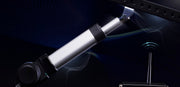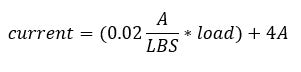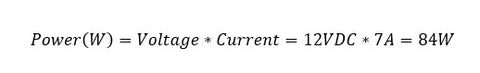# Calculating Power Consumption of Electric Linear ActuatorsPA Engineer

Power. Wattage. Energy Consumption. All these terms can be used when discussing electric linear actuator power consumption. But what exactly do they mean and how do you calculate the power consumption of a 12V DC motor or any motor/linear actuator? That is exactly what we intend to show you in this article.

## Current Draw of Linear Actuators

Electric linear actuators that are manufactured by Progressive Automations use DC motors. Depending on the force that the linear actuator can apply, the size of the DC motor will vary. The higher the force, the larger the DC motor.

DC motors with a low current draw will typically be used in the actuator with a lower force rating – the same goes for a DC motor with a higher current draw for actuators with higher force ratings. Current draw ratings are provided as a threshold for the maximum current that the DC motor will draw when applying a force equal to the rated force of an actuator.

For example, an in-stock PA-04-400LB model has a 400lbf load and 12A current draw rating. In this case, when 400lbf is applied to the actuator, the current draw will be 12A with a 12VDC input. Although 12A at 400lbs is the rated value, the use case of the actuator in your application may result in a lower force being applied. However, the current draw of the rest of your system (i.e., control box, active sensors, etc.) could result in a higher overall system current draw, which means the DC motor power consumption will be higher.

## Data Interpolation

Let’s run a scenario where a force of 150lbf is applied onto the PA-04-400LB model to see the current draw reading from a graph. Below is a load versus current graph. We will need to create an equation to interpolate the current draw at a specified force rating.The no-load current is 4A and the full-load current is 12A. What we see is a direct linear relationship between an increase in load and an increase in current draw. Since this is a linear relationship, we can apply a straight-line formula:

y = mx + b

Where,

y = Current (A) at 12VDC

m = Slope of the 400lbs PA-04 current versus load line

b = No-load current draw of the PA-04

Since we have all the values for y, b, we need to determine m. To find m, we need to select two arbitrary points on the slope. We will use the no-load and full-load values for y and x. Use the equation below to determine m.Now, we can calculate the current draw at 150lbs of force by plugging it into the straight-line formula.When you use the above equation with a load of 150lbs, the current draw calculates to 7A.

## Calculating Power Consumption

The power consumption of your linear actuator based on ideal and actual values may vary due to external factors such as:

• Ambient temperature.
• Operating conditions.
• Variance in motor build.
• Use of third-party control equipment.
• Actual applied force.

### Ideal Power Consumption

The basic equation to calculate power consumption is as follows:

Power = Voltage * Current

If we want to calculate the power consumption of the PA-04-400LBS when a 150lbs load is applied, we can plug the calculated current draw of 7A into the equation.Using the power consumption formula above, the calculated power draw at 150LB for the PA-04-400LB is 84W. What this means is that you can compare various models or multiple linear actuators within a system to determine the power consumption and whether it fits the requirements of your application. If not, you can always decrease the load, opt for a power-efficient linear actuator, or reduce your overall system current draw. However, the power value calculated above is theoretical. So, let’s calculate a true measurement.

### Actual Power Consumption

To obtain a true measurement of the linear actuator power consumption under continuous motion with a fixed applied load, you will need a voltmeter and an ammeter to measure the actual input voltage and current draw.

For example, the input voltage may be 12.2VDC and the current draw may be 7.4A. In this circumstance, the power consumption will be 90.3W (when using the power consumption formula described above). Comparing the actual versus the ideal current draw, we can determine the percentage change between the two values.

Actual Power Consumption = 90.3W

Ideal Power Consumption = 84W

For a difference of 6.3W, there is a 7.6% power increase based on the ideal value when compared to the actual value.